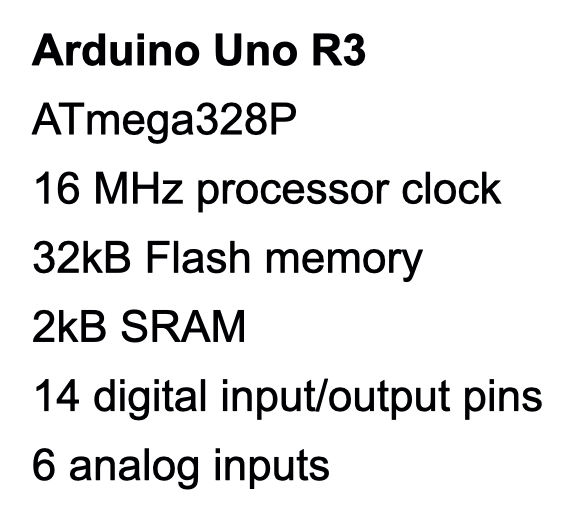top of page
Search

# Arduino CW decoder project part 1

Updated: Oct 9, 2021

Since completing the oscilloscope project I have been working on a CW (Morse code) decoder. After trying 5 different designs and techniques I have finally homed in on a solution that works well. This video is an early prototype decoding a Titanic message video on YouTube.

Using the Arduino as a starting point meant I could use the knowledge of hardware and software that I had developed whilst working on the oscilloscope. Some of the issues I encountered could have been mitigated by using more processing power but I was enjoying the challenge of working around the limitations of memory and speed so I persevered with the Arduino.Arduino Uno R3 spec

The Arduino Uno

To recap, the Arduino Uno R3 specification is outlined in the text box but the main limitations for my projects were:

• The analog to digital converter (ADC) is relatively slow.

• Driving displays takes a lot of time and memory (especially if using the published libraries)

• 2kB of RAM is very restrictive for processing dynamic data.

With this in mind, my initial thought was to reduce the processing load by using hardware to separate the signal from the carrier. After some simulation and testing of low pass filters, I decided that this approach was not feasible and would result in too much distortion of the wanted signal.Audio Sampling

The next step was to feed a sample CW signal into the Arduino ADC for software processing. To do this I used a MAX9814 microphone module and a simple op amp circuit to amplify the signal.

For sample signals I turned to YouTube where there is a wide range of training videos and actual CW contact recordings.

My Arduino oscilloscope allowed me to visualise the signal but I also used a new feature of the Arduino IDE - a serial link plot function. This runs on the host computer and takes the serial print output of the Arduino and plots it on a chart rather than just printing it out. However, at this point the limitations of the Arduino became apparent.

The ADC takes around 15 μs and serial print takes over 100 μs to print an integer. This limits a real time display to a maximum frequency of around 2kHz (using a sampling rate of twice the signal frequency).

Alternatively, the samples can be stored in an array and printed out in batches. This allows frequencies up to around 12kHz to be displayed but the 2kB ram can only store about 500 samples. Still, working between these limits with suitable triggering gave a useful view of the signal to be processed. This was invaluable for fault finding and diagnostics.

Signal Processing

A simple analysis of the analog signal samples was sufficient to identify when the carrier was present but this approach was easily defeated by background noise and changes in volume. Clearly a better solution would be to digitally extract the amplitude of the carrier frequency which pointed me in the direction of the Discrete Fourier Transform (DFT).

I spent a lot of time finding out how DFT worked and simulating it on Excel to find the best combination of sampling frequency and number of samples. The number of samples equals the number of "bins" for the frequency amplitude measurements. Each bin is a centre frequency for which the amplitude can be captured. Only half the bins can be used (ie sampling frequency must be at least twice the target frequency. However the number of samples also determines the accuracy of the measurements.

The Fourier calculations can be simplified by solving for a single target frequency using the Goertzel algorithm and ignoring the imaginary part of the signal. This worked equally well in the simulation and was much easier to process on the Arduino.

To use DFT on a waveform it needs to have positive and negative values about a zero reference point. The Arduino ADC works with a positive voltage and gives a value from 0 - 1023. This was resolved by taking the average value of the waveform over time and using that value as the zero reference point. This was then subtracted from each sample before the DFT processing.

I started with around 11,500 samples per second and 50 samples which gives a measurement time of 4.5ms snd a bin size of 230Hz. Although this worked well in the Excel simulation, I couldn't replicate the results on the Arduino and I eventually realised that the floating point calculations were taking too long and disrupting the sampling speed. To solve this I took the sampling rate down to 4,800 samples per second and used 16 samples to give a measurement time of 3.3ms and a bin size of 300Hz.This configuration gave a workable CW signal that I could now start decoding . I will pick this up in the next post.

Further information

By far the best description of DFT that I found was an article in Practical Cryptograph:

The Goertzel algorithm is explained in Wikipedia:

https://en.wikipedia.org/wiki/Goertzel_algorithm